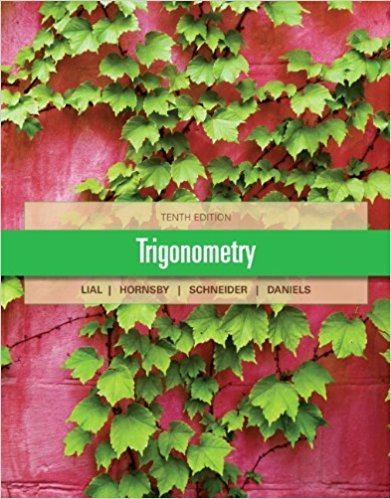> > > Chapter 2.5

# Solutions for Chapter 2.5: Further Applications of Right Triangles## Full solutions for Trigonometry | 10th Edition

ISBN: 9780321671776Solutions for Chapter 2.5: Further Applications of Right Triangles

Solutions for Chapter 2.5
4 5 0 249 Reviews
11
0
##### ISBN: 9780321671776

Since 44 problems in chapter 2.5: Further Applications of Right Triangles have been answered, more than 18047 students have viewed full step-by-step solutions from this chapter. Trigonometry was written by and is associated to the ISBN: 9780321671776. This expansive textbook survival guide covers the following chapters and their solutions. Chapter 2.5: Further Applications of Right Triangles includes 44 full step-by-step solutions. This textbook survival guide was created for the textbook: Trigonometry, edition: 10.

Key Math Terms and definitions covered in this textbook
• Back substitution.

Upper triangular systems are solved in reverse order Xn to Xl.

• Cholesky factorization

A = CTC = (L.J]))(L.J]))T for positive definite A.

• Commuting matrices AB = BA.

If diagonalizable, they share n eigenvectors.

• Companion matrix.

Put CI, ... ,Cn in row n and put n - 1 ones just above the main diagonal. Then det(A - AI) = ±(CI + c2A + C3A 2 + .•. + cnA n-l - An).

• Diagonalization

A = S-1 AS. A = eigenvalue matrix and S = eigenvector matrix of A. A must have n independent eigenvectors to make S invertible. All Ak = SA k S-I.

• Fast Fourier Transform (FFT).

A factorization of the Fourier matrix Fn into e = log2 n matrices Si times a permutation. Each Si needs only nl2 multiplications, so Fnx and Fn-1c can be computed with ne/2 multiplications. Revolutionary.

• Incidence matrix of a directed graph.

The m by n edge-node incidence matrix has a row for each edge (node i to node j), with entries -1 and 1 in columns i and j .

• Kronecker product (tensor product) A ® B.

Blocks aij B, eigenvalues Ap(A)Aq(B).

• Lucas numbers

Ln = 2,J, 3, 4, ... satisfy Ln = L n- l +Ln- 2 = A1 +A~, with AI, A2 = (1 ± -/5)/2 from the Fibonacci matrix U~]' Compare Lo = 2 with Fo = O.

• Multiplier eij.

The pivot row j is multiplied by eij and subtracted from row i to eliminate the i, j entry: eij = (entry to eliminate) / (jth pivot).

• Nullspace N (A)

= All solutions to Ax = O. Dimension n - r = (# columns) - rank.

• Polar decomposition A = Q H.

Orthogonal Q times positive (semi)definite H.

• Positive definite matrix A.

Symmetric matrix with positive eigenvalues and positive pivots. Definition: x T Ax > 0 unless x = O. Then A = LDLT with diag(D» O.

• Rank r (A)

= number of pivots = dimension of column space = dimension of row space.

• Spectral Theorem A = QAQT.

Real symmetric A has real A'S and orthonormal q's.

• Toeplitz matrix.

Constant down each diagonal = time-invariant (shift-invariant) filter.

• Trace of A

= sum of diagonal entries = sum of eigenvalues of A. Tr AB = Tr BA.

• Tridiagonal matrix T: tij = 0 if Ii - j I > 1.

T- 1 has rank 1 above and below diagonal.

v + w = (VI + WI, ... , Vn + Wn ) = diagonal of parallelogram.

• Vector space V.

Set of vectors such that all combinations cv + d w remain within V. Eight required rules are given in Section 3.1 for scalars c, d and vectors v, w.

×

I don't want to reset my password

Need help? Contact support

Need an Account? Is not associated with an account
We're here to help